HomeBig KidLearning & EducationSubtraction For Preschoolers And Kids

# Subtraction For Preschoolers And Kids

Does your child know how to do addition and wonder what’s next? Subtraction is the next mathematical operation that you must teach your little one. In this article, we have covered the topic of how to teach subtraction to kids, examples and some word problems to help your child understand the same.

## What Is Subtraction?

In maths, subtraction refers to an operation that is used to find the difference between numbers. When you take away a few objects from a group of objects, the group becomes smaller. Therefore, we subtracted a few objects from a group of objects. When we subtract something, we denote it with the help of the minus sign “-“. Let’s take a look at this image to understand the practical application of subtraction.

###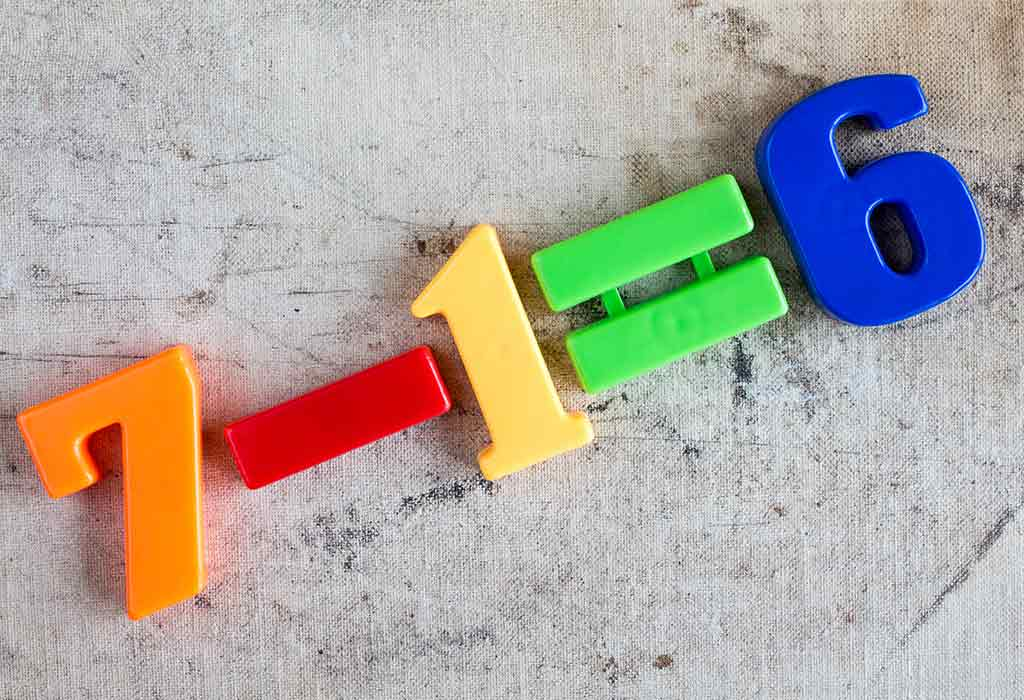For example, you bought 7 chocolates for your birthday party, and your friends ate 1 chocolate. Now you are left with 6 chocolates. This can be written as: 7 – 1 = 6 and is read as “seven minus one equals six”. When we subtract 1 from 7, (7 – 1) we get 6. Here, we have performed the subtraction operation on two numbers 7 and 1, to get the difference of 6.

## When Should Your Child Learn Subtraction?

Alongside addition, subtraction is an important mathematical concept that is taught to children in first grade. They are taught to solve advanced subtraction problems gradually. Subtraction helps children to understand to associate numbers with objects and calculate and express losses using math language.

## Symbol Of Subtraction

We use different symbols to represent different operations in maths. For subtraction, we use the minus sign or “-” between the two numbers to represent that we are going to perform subtraction. For example, in the expression “5 – 2 = 3”, the symbol “-” connects the two numbers and tells us that we have to perform the subtraction between the two numbers.

## What Is The Formula Of Subtraction?

###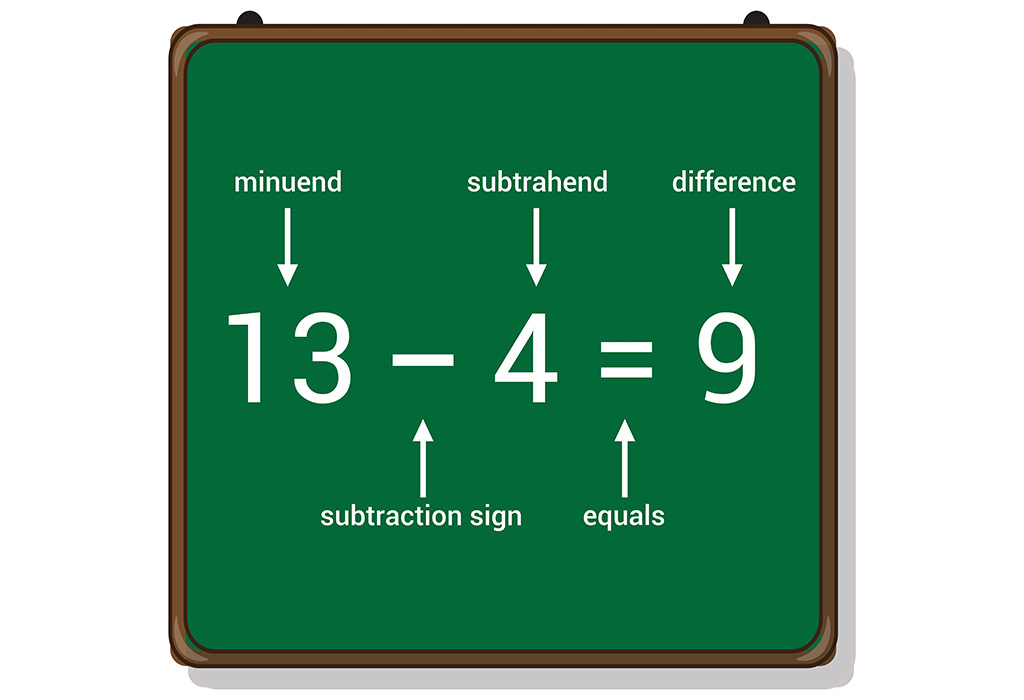The above image will help you understand the formula of subtraction. The minuend, subtrahend, and difference are parts of a subtraction expression. Where,

• The minuend is the number from which the other number is subtracted.
• The subtrahend is the number which is to be subtracted from the minuend.
• The difference is the final result after subtracting the subtrahend from the minuend.

Therefore, the subtraction formula: Minuend – Subtrahend = Difference

In the subtraction problem in the image, 13 – 4 = 9, the number 13 is the minuend, the number 4 is the subtrahend, and the number 9 is the difference.

## What Are The Properties of Subtraction?

Let’s take a look at the properties of subtraction for kids:

• Subtraction is the reverse of addition.
• When we subtract a number from itself, it gives the answer 0.
• For any two whole numbers, a and b, if a > b, then a – b is a whole number and if a < b, then a – b is never a whole number.
• Subtraction of whole numbers of not communicative. Therefore, any two whole numbers, a and b, a – b ≠ b – a.
• Subtraction of whole numbers if not associative. Therefore, for any three whole numbers, say a, b, and c, (a – b) – c ≠ a – (b – c).

## Subtraction For Preschoolers With Examples

To make it easy for your little one to understand subtraction, we have covered some examples below, along with the image. Let’s read and solve!

• 5 – 2 = 3
• 6 – 4 = 2
• 4 – 3 = 1

## Subtraction For Lower Elementary Kids With Examples

In this section, we will learn the subtraction of two-digit numbers, three-digit numbers, and four-digit numbers, with and without regrouping, with the help of examples.

### Subtraction Of Two Digit Numbers

Let’s take a look at 2-digit subtraction for classes 1, 2 and 3.

#### 1. Subtract 40 from 78.

1. Line up the numbers according to place value. Place the bigger number on top.
2. In the ones column, we have 8 and O.
3. In the tens column, we have 7 and 4.
4. Solve the digit at the ones place. (8 – 0 = 8)
5. Next, solve the digits at the tens place. (7 – 4 = 3)
6. So, the difference between the two given numbers is: 78 – 40 = 38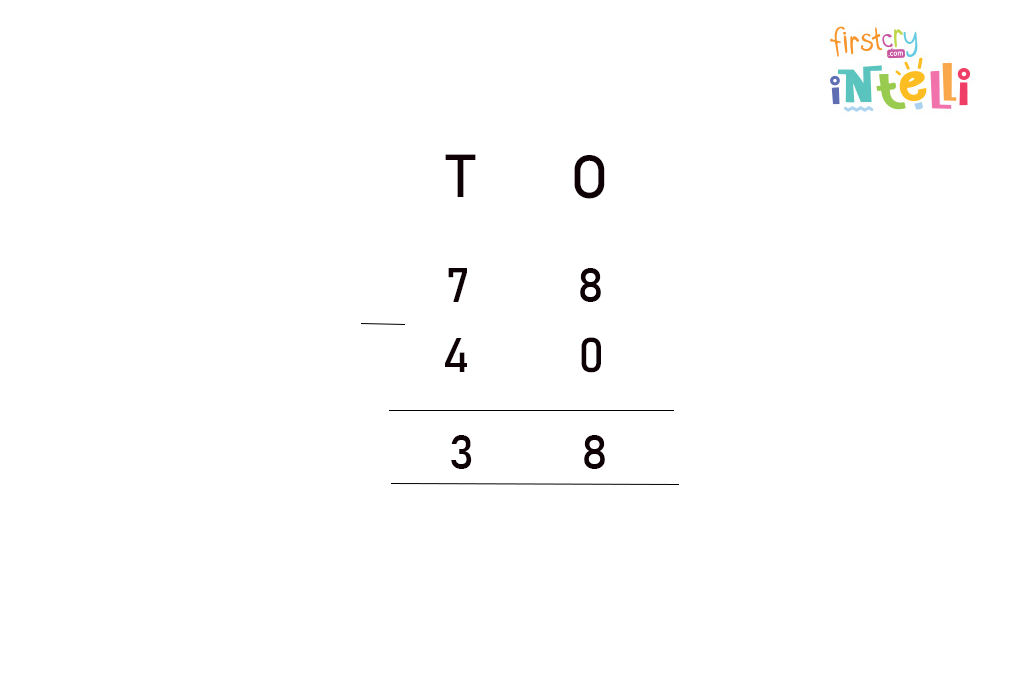### Subtraction Of Three Digit Numbers

Let’s take a look at 3-digit subtraction for classes 1, 2 and 3.

#### 1. Subtract 140 from 250.

1. Line up the numbers according to place value. Place the bigger number on top.
2. In the ones column, we have 0 and O.
3. In the tens column, we have 5 and 4.
4. In the hundreds column, we have 2 and 1.
5. Solve the digit at the ones place. (0 – 0 = 0)
6. Next, solve the digits at the tens place. (5 – 4 = 1)
7. Finally, solve the digits at the hundreds place (2 – 1 = 1)
8. So, the difference between the given numbers is: 250 – 140 = 110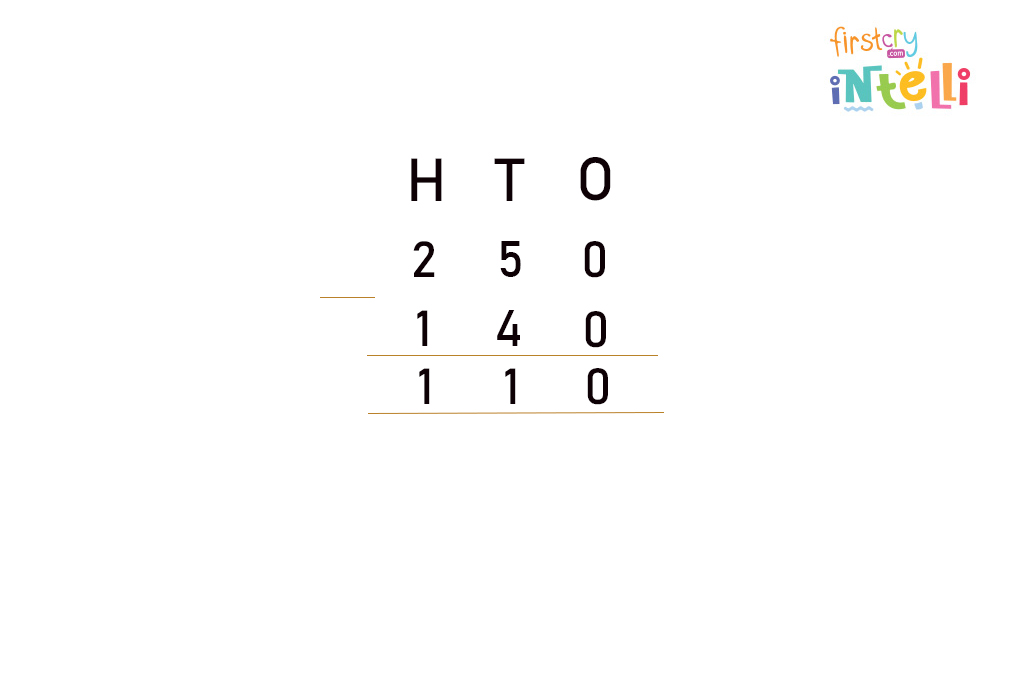### Subtraction Of Four Digit Numbers

Let’s take a look at 4-digit subtraction for classes 1, 2 and 3.

#### 1. Subtract 1423 from 2764

1. Line up the numbers according to place value. Place the bigger number on top.
2. In the ones column, we have 4 and 3.
3. In the tens column, we have 6 and 2.
4. In the hundreds column, we have 7 and 4.
5. In the thousands column, we have 2 and 1.
6. Solve the digit at the ones place. (4 – 3 = 1)
7. Next, solve the digits at the tens place. (6 – 2 = 4)
8. Solve the digits at the hundreds place (7 – 4 = 3)
9. Finally, solve the digits at thousands place (2 –1 =1)
10. So, the difference between the given numbers is: 2764 – 1423 = 1341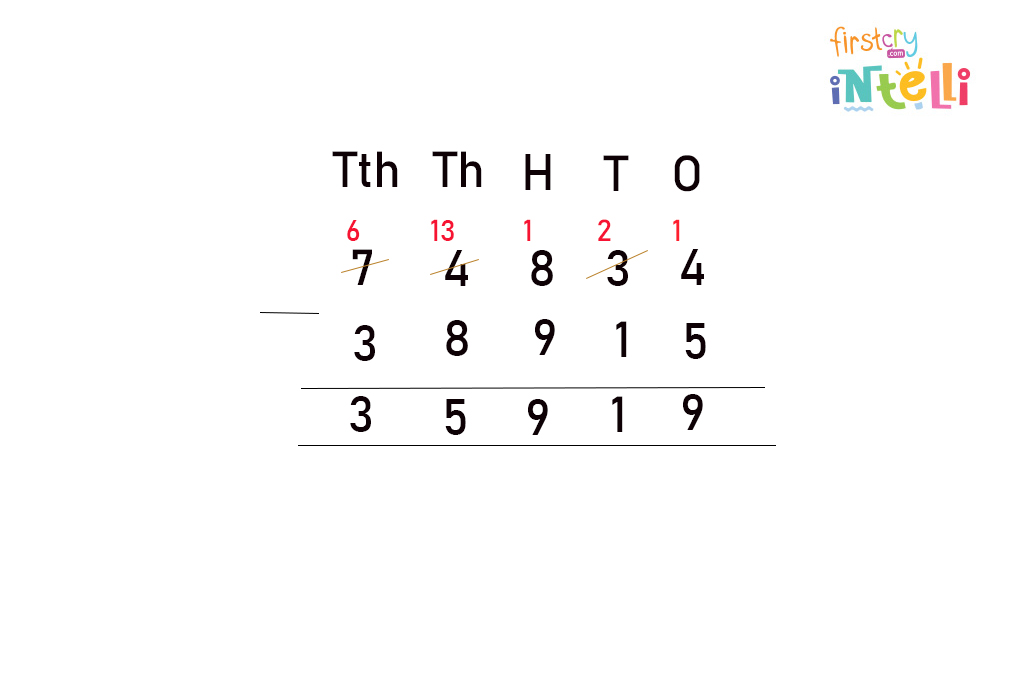### Subtract Without Regrouping

In this section, we will take a look at how to do subtraction without borrowing.

#### 1. Subtract 2743 from 3745.

1. Solve the digit at the ones place. (5 – 3 = 2)
2. Next, solve the digits at the tens place. (4 – 4 = 0)
3. Solve the digits at the hundreds place (7 – 7 = 0)
4. Finally, solve the digits at thousands place (3 –2 =1)
5. So, the difference between the given numbers is: 3745 – 2743 = 1002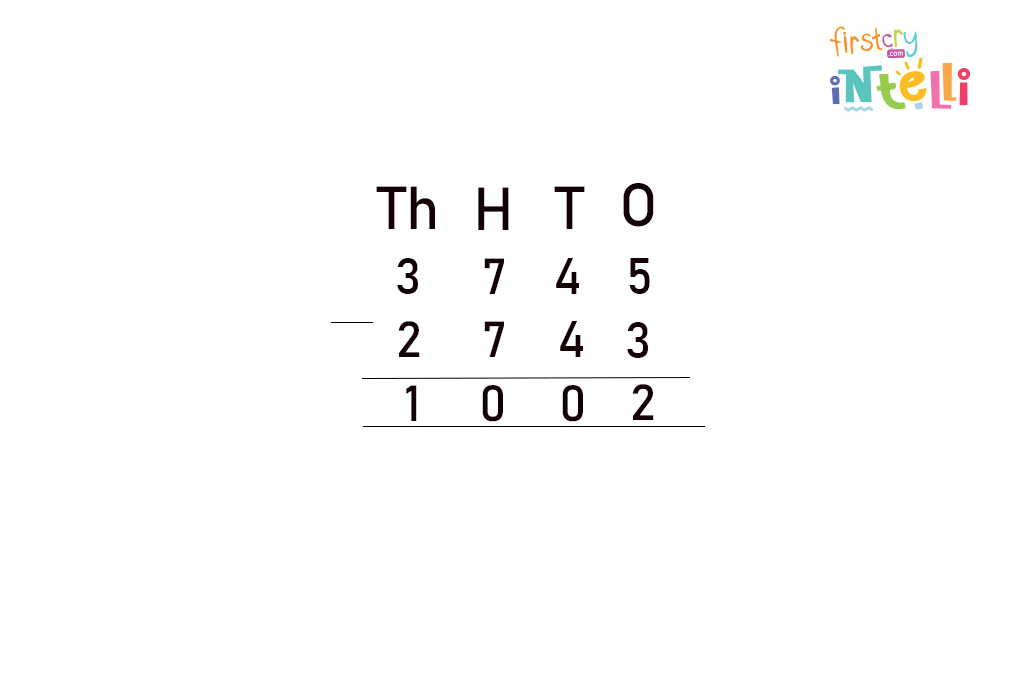### Subtract With Regrouping

In this section, we will take a look at how to do subtraction with borrowing.

#### 1. Subtract 3186 from 4562.

1. Solve the digits at the ones place. Since 6 is greater than 2, we will borrow 1 from the tens place, and 2 will become 12. (12 – 6 = 6)
2. As 6 donated 1 to the ones place, it will become 5. Here 8 is greater than 5, so we will borrow 1 from hundreds place, and 5 will become 15. (15 – 8 = 7)
3. As 5 donated 1 to the tens place, we have 4 at hundreds place now. (4 – 1 = 3)
4. Finally, we will subtract the digits at the thousands place. (4 – 3 = 1)
5. So, the difference between the given numbers is: 4562 – 3186 = 1376

## How To Subtract Using Number Line?

Let’s learn how to do subtraction on a number line.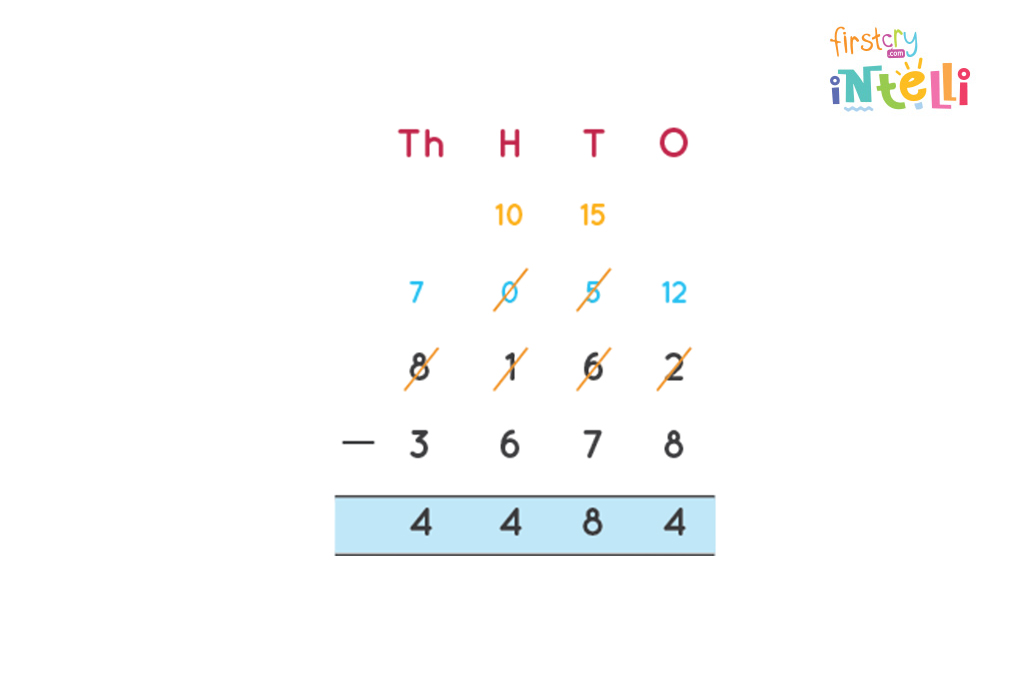A number line enables us to jump backwards and forward on each number and makes it easy to understand subtraction. For example, to subtract 4 from 9 using a number line, we will first mark 9 on the number line. Now, we have to subtract 4 from 9. Therefore, we will move 4 times to the left on a number line. The number on which your will land will be 5. So, 9 – 4 = 5.

## Practice Problems On Subtraction For Kids

Here are a few subtraction word problems for kids to practice. This will help children to strengthen their problem-solving skills and also understand how to do subtraction.

1. Harry collected 180 seashells, and Emily collected 50 shells. Who collected more shells, and by how much?

Solution:
Number of shells collected by Harry = 180
Number of shells collected by Emily = 50
H T O
1 8 0
–  5 0
_____
1 3 0|
Therefore, Harry collected 130 seashells more than Emily.

2. In a cricket match, India scored 236 runs, and Australia scored 126 runs. How many more runs should Australia score to be equal to India’s score?

Solution:

Runs scored by India = 236
Runs scored by Australia = 126
To find the number of runs that Australia should score more to be equal to the number of runs scored by India, we will subtract 126 from 236.
H T O
2 3 6
– 1 2 6
______
1 1 0
Therefore, Australia must score 110 more runs to be equal to India’s runs.

## FAQs

### 1. What Are The Rules For Subtracting?

Here are the rules for subtracting:

We have to take one number away from another. So, if we have 5 toffees and eat 4 of them, we’ll have 1 toffee left. That means we subtracted 4 toffees from the original 5 toffees total to get the final 1 toffee total.

### 2. What Are The Other Names For Subtraction?

The other names for subtraction are as follows:

1. Difference
2. Less than
3. Leftover
4. Deficit
5. Shortage
6. Shortfall
7. Surplus

### 3. What Are The Facts About Subtraction?

Here are some facts about subtraction:

• Subtraction is nothing but the opposite of addition.
• Subtracting zero from a number doesn’t change its value.

### 4. What Are The Three Parts of subtraction?

The three parts of subtraction are:

• Minuend is the number from which the other number is subtracted.
• Subtrahend is the number which is to be subtracted from the minuend.
• Difference is the final result after subtracting the subtrahend from the minuend.

We hope this article helped your little one to learn how to subtract. After this, you can introduce your little one to multiplication and division.

RELATED ARTICLES